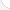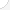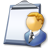Computer software engineersDescription: Computer software engineers do research, design computers, and find new ways to use them in business. They may identify problems in business, science, and engineering. Then they use computers to solve the problems. This kind of work can result in eyestrain, backaches, and hand and wrist problems.Source: Bureau of Labor Statistics, U.S. Department of Labor, Occupational Outlook Handbook, 2008-09 Edition at http://www.bls.gov/OCO/Complete Job Profile: http://www.bls.gov/oco/ocos267.htmSalary: \$50,001 or more per yearComments:I've used so much math lately it makes me wish I paid more attention in high school. Software engineer again. In this past month alone I've had to 1. attempt to calculate how much I can push my program before it runs out of memory (that's somewhat basic) 1.5 refresh my memory on the modulus operator 2. look up polynominals and cyclical record check to better understand the hash table I've been working with 3. and the worst one of all, I need to determine how to calculate the best 12 number combination set from a set of 20 numbers ranging from 1 to 80 in over 600,000 records. And due to memory & time limitations I can only calculate the best 4 number sets. So I'm trying to determine if I took all the records with the best 4 number sets and figured out the best combinations within those sets (with out the 4 number set) whether those would in the end be the best 12 number set. I don' think it would be, I think I'd have the best 12 number set within the best 4 number sets which wouldn't be the same.

There are 68 math topics Computer software engineers need to know.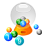Basic Math / Algebra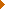FractionsDecimalsRatio and ProportionPercentCustomary MeasurementMetric MeasurementMeasurement ConversionBasic ProbabilityBasic StatisticsStatistical GraphingPowers and RootsOther Number BasesNegative NumbersScientific NotationBasic Problem Solving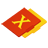First-Year AlgebraUsing FormulasLinear EquationsLinear InequalitiesOperations with PolynomialsFactoring PolynomialsRational ExpressionsCoordinate Graphing 2DLinear SystemsQuadratic EquationsAlgebraic Representation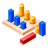GeometryBasic TerminologyAngle MeasurementCongruent TrianglesTriangle InequalitiesParallel LinesQuadrilateralsSimilarityPythagorean TheoremRight Triangle TrigonometryCirclesAreaVolumeTransformationsMake/Use 3D Drawings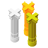Second-Year Algebra / TrigonometryFunctionsVariationImaginary NumbersPolynomial EquationsLogarithmsSequences and SeriesMatricesCoordinate Graphing 3DAdvanced ProbabilityAdvanced StatisticsConic SectionsNon-Linear SystemsTrigonometric/Circular FunctionsGraphs of Trigonometric FunctionsTrigonometric IdentitiesTrigonometric Equations/InversesOblique TrianglesPolar Coordinates/GraphsVectors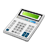Other TopicsCalculus and Higher MathBasic Calculator UseScientific Calculator UseComputer UseComputer ProgrammingGroup Problem SolvingMental MathInductive/Deductive ReasoningMath CommunicationsMathematical Modeling